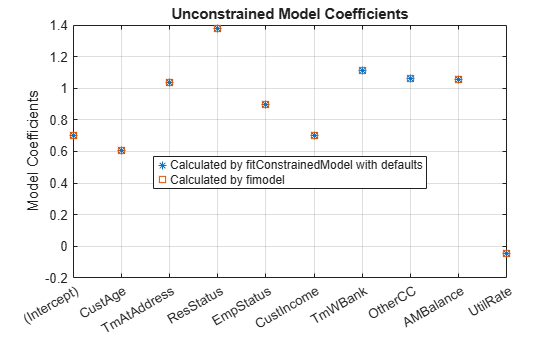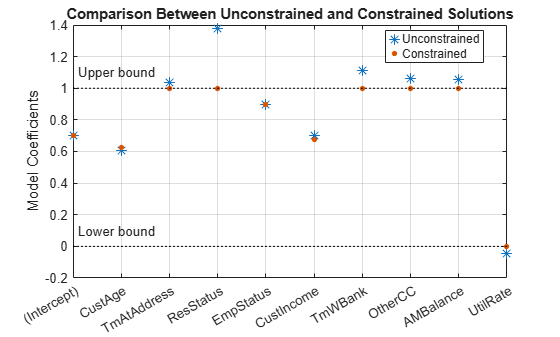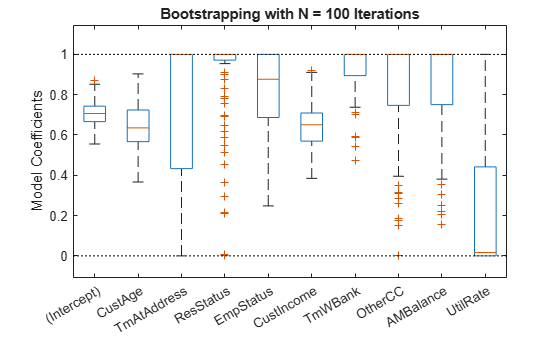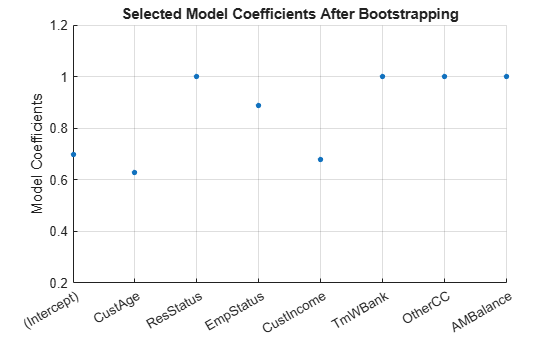# fitConstrainedModel

Fit logistic regression model to Weight of Evidence (WOE) data subject to constraints on model coefficients

## Syntax

``[sc,mdl] = fitConstrainedModel(sc)``
``````[sc,mdl] = fitConstrainedModel(___,Name,Value)``````

## Description

example

````[sc,mdl] = fitConstrainedModel(sc)` fits a logistic regression model to the Weight of Evidence (WOE) data subject to equality, inequality, or bound constraints on the model coefficients. `fitConstrainedModel` stores the model predictor names and corresponding coefficients in an updated `creditscorecard` object `sc` and returns the `GeneralizedLinearModel` object `mdl` which contains the fitted model. ```

example

``````[sc,mdl] = fitConstrainedModel(___,Name,Value)``` specifies options using one or more name-value pair arguments in addition to the input arguments in the previous syntax.```

## Examples

collapse all

To compute scores for a `creditscorecard` object with constraints for equality, inequality, or bounds on the coefficients of the logistic regression model, use `fitConstrainedModel`. Unlike `fitmodel`, `fitConstrainedModel` solves for both the unconstrained and constrained problem. The current solver used to minimize an objective function for `fitConstrainedModel` is `fmincon`, from the Optimization Toolbox™.

This example has three main sections. First, `fitConstrainedModel` is used to solve for the coefficients in the unconstrained model. Then, `fitConstrainedModel` demonstrates how to use several types of constraints. Finally, `fitConstrainedModel` uses bootstrapping for the significance analysis to determine which predictors to reject from the model.

Create the `creditscorecard` Object and Bin data

```load CreditCardData.mat sc = creditscorecard(data,'IDVar','CustID'); sc = autobinning(sc);```

Unconstrained Model Using `fitConstrainedModel`

Solve for the unconstrained coefficients using `fitConstrainedModel` with default values for the input parameters. `fitConstrainedModel` uses the internal optimization solver `fmincon` from the Optimization Toolbox™. If you do not set any constraints, `fmincon` treats the model as an unconstrained optimization problem. The default parameters for the `LowerBound` and `UpperBound` are `-Inf` and `+Inf`, respectively. For the equality and inequality constraints, the default is an empty numeric array.

```[sc1,mdl1] = fitConstrainedModel(sc); coeff1 = mdl1.Coefficients.Estimate; disp(mdl1.Coefficients);```
``` Estimate _________ (Intercept) 0.70246 CustAge 0.6057 TmAtAddress 1.0381 ResStatus 1.3794 EmpStatus 0.89648 CustIncome 0.70179 TmWBank 1.1132 OtherCC 1.0598 AMBalance 1.0572 UtilRate -0.047597 ```

Unlike `fitmodel` which gives p-values, when using `fitConstrainedModel`, you must use bootstrapping to find out which predictors are rejected from the model, when subject to constraints. This is illustrated in the "Significance Bootstrapping" section.

Using `fitmodel` to Compare the Results and Calibrate the Model

`fitmodel` fits a logistic regression model to the Weight-of-Evidence (WOE) data and there are no constraints. You can compare the results from the "Unconstrained Model Using fitConstrainedModel" section with those of `fitmodel` to verify that the model is well calibrated.

Now, solve the unconstrained problem by using `fitmodel`. Note that `fitmodel` and `fitConstrainedModel` use different solvers. While `fitConstrainedModel` uses `fmincon`, `fitmodel` uses `stepwiseglm` by default. To include all predictors from the start, set the `'VariableSelection'` name-value pair argument of `fitmodel` to `'fullmodel'`.

```[sc2,mdl2] = fitmodel(sc,'VariableSelection','fullmodel','Display','off'); coeff2 = mdl2.Coefficients.Estimate; disp(mdl2.Coefficients);```
``` Estimate SE tStat pValue _________ ________ _________ __________ (Intercept) 0.70246 0.064039 10.969 5.3719e-28 CustAge 0.6057 0.24934 2.4292 0.015131 TmAtAddress 1.0381 0.94042 1.1039 0.26963 ResStatus 1.3794 0.6526 2.1137 0.034538 EmpStatus 0.89648 0.29339 3.0556 0.0022458 CustIncome 0.70179 0.21866 3.2095 0.0013295 TmWBank 1.1132 0.23346 4.7683 1.8579e-06 OtherCC 1.0598 0.53005 1.9994 0.045568 AMBalance 1.0572 0.36601 2.8884 0.0038718 UtilRate -0.047597 0.61133 -0.077858 0.93794 ```
```figure plot(coeff1,'*') hold on plot(coeff2,'s') xticklabels(mdl1.Coefficients.Properties.RowNames) ylabel('Model Coefficients') title('Unconstrained Model Coefficients') legend({'Calculated by fitConstrainedModel with defaults','Calculated by fimodel'},'Location','best') grid on```As both the tables and the plot show, the model coefficients match. You can be confident that this implementation of `fitConstrainedModel` is well calibrated.

Constrained Model

In the constrained model approach, you solve for the values of the coefficients ${b}_{i}$ of the logistic model, subject to constraints. The supported constraints are bound, equality, or inequality. The coefficients maximize the likelihood-of-default function defined, for observation $\mathit{i}$, as:

`${\mathit{L}}_{\mathit{i}}={\mathit{p}\left({\mathrm{Default}}_{\mathit{i}}\right)}^{{\mathit{y}}_{\mathit{i}}}×{\left(1-\mathit{p}\left({\mathrm{Default}}_{\mathit{i}}\right)\right)}^{1-{\mathit{y}}_{\mathit{i}}}\text{\hspace{0.17em}\hspace{0.17em}\hspace{0.17em}\hspace{0.17em}\hspace{0.17em}\hspace{0.17em}\hspace{0.17em}\hspace{0.17em}\hspace{0.17em}\hspace{0.17em}\hspace{0.17em}\hspace{0.17em}\hspace{0.17em}\hspace{0.17em}\hspace{0.17em}\hspace{0.17em}\hspace{0.17em}\hspace{0.17em}\hspace{0.17em}\hspace{0.17em}}$`

where:

• ${\mathit{p}\left({\mathrm{Default}}_{\mathit{i}}\right)=\frac{\text{\hspace{0.17em}}\text{\hspace{0.17em}}\text{\hspace{0.17em}}\text{\hspace{0.17em}}1\text{\hspace{0.17em}\hspace{0.17em}\hspace{0.17em}\hspace{0.17em}}}{1+{\mathit{e}}^{-\mathit{b}{\mathrm{x}}_{\mathit{i}}}}\text{\hspace{0.17em}}}^{\text{\hspace{0.17em}}}$

• $\mathbit{b}=\left[{\mathit{b}}_{1\text{\hspace{0.17em}}}{\mathit{b}}_{2}\text{\hspace{0.17em}}...{\mathit{b}}_{\mathit{K}}\right]$ is an unknown model coefficient

• ${\mathbit{x}}_{\mathbit{i}}=\left[{\mathit{x}}_{\mathrm{i1}}\text{\hspace{0.17em}}{\mathit{x}}_{2}\text{\hspace{0.17em}}...{\mathit{x}}_{\mathrm{iK}}\right]$ is the predictor values at observation $\mathit{i}$

• ${\mathit{y}}_{\mathit{i}}$ is the response value; a value of 1 represents default and a value of 0 represents non-default

This formula is for non-weighted data. When observation i has weight ${\mathit{w}}_{\mathit{i}}$, it means that there are ${\mathit{w}}_{\mathit{i}}$ as many observations i. Therefore, the probability that default occurs at observation i is the product of the probabilities of default:

`${\mathit{p}}_{\mathit{i}\text{\hspace{0.17em}}}=\text{\hspace{0.17em}}\underset{{\mathit{w}}_{\mathit{i}\text{\hspace{0.17em}}}\mathrm{times}}{{\mathit{p}\left({\mathrm{Default}}_{\mathit{i}}\right)}^{{\mathit{y}}_{\mathit{i}}}*{\mathit{p}\left({\mathrm{Default}}_{\mathit{i}}\right)}^{{\mathit{y}}_{\mathit{i}}}*...*{\mathit{p}\left({\mathrm{Default}}_{\mathit{i}}\right)}^{{\mathit{y}}_{\mathit{i}}}}={\mathit{p}\left({\mathrm{Default}}_{\mathit{i}}\right)}^{{{\mathit{w}}_{\mathit{i}}*\mathit{y}}_{\mathit{i}}}$`

Likewise, the probability of non-default for weighted observation i is:

`${\stackrel{ˆ}{\mathit{p}}}_{\mathit{i}\text{\hspace{0.17em}}}=\text{\hspace{0.17em}}\underset{{\mathit{w}}_{\mathit{i}\text{\hspace{0.17em}}}\mathrm{times}}{{\mathit{p}\left({~\mathrm{Default}}_{\mathit{i}}\right)}^{{1-\mathit{y}}_{\mathit{i}}}*{\mathit{p}\left(~{\mathrm{Default}}_{\mathit{i}}\right)}^{1-{\mathit{y}}_{\mathit{i}}}*...*{\mathit{p}\left(~{\mathrm{Default}}_{\mathit{i}}\right)}^{1-{\mathit{y}}_{\mathit{i}}}}={\left(1-\mathit{p}\left({\mathrm{Default}}_{\mathit{i}}\right)\right)}^{{\mathit{w}}_{\mathit{i}}*\left(1-{\mathit{y}}_{\mathit{i}}\right)}$`

For weighted data, if there is default at a given observation i whose weight is ${\mathit{w}}_{\mathit{i}}$, it is as if there was a ${\mathit{w}}_{\mathit{i}}$ count of that one observation, and all of them either all default, or all non-default. ${\mathit{w}}_{\mathit{i}}$ may or may not be an integer.

Therefore, for the weighted data, the likelihood-of-default function for observation i in the first equation becomes

`${\mathit{L}}_{\mathit{i}}={\mathit{p}\left({\mathrm{Default}}_{\mathit{i}}\right)}^{{{\mathit{w}}_{\mathit{i}}*\mathit{y}}_{\mathit{i}}}×{\left(1-\mathit{p}\left({\mathrm{Default}}_{\mathit{i}}\right)\right)}^{{\mathit{w}}_{\mathit{i}}*\left(1-{\mathit{y}}_{\mathit{i}}\right)}$`

By assumption, all defaults are independent events, so the objective function is

`$\mathit{L}={\mathit{L}}_{1}×{\mathit{L}}_{2}×...×{\text{\hspace{0.17em}}\mathit{L}}_{\mathit{N}}$`

or, in more convenient logarithmic terms:

`$\mathrm{log}\left(\mathit{L}\right)={\sum }_{\mathit{i}=1}^{\mathit{N}}{\mathit{w}}_{\mathit{i}}*\left[{\mathit{y}}_{\mathit{i}}\mathrm{log}\left(\mathit{p}\left({\mathrm{Default}}_{\mathit{i}}\right)\right)+\left(1-{\mathit{y}}_{\mathit{i}}\right)\mathrm{log}\left(1-\mathit{p}\left({\mathrm{Default}}_{\mathit{i}}\right)\right)\right]\text{\hspace{0.17em}}$`

Apply Constraints on the Coefficients

After calibrating the unconstrained model as described in the "Unconstrained Model Using fitConstrainedModel" section, you can solve for the model coefficients subject to constraints. You can choose lower and upper bounds such that $0\le {\mathit{b}}_{\mathit{i}}\le 1,\forall \mathit{i}=1...\mathit{K}$, except for the intercept. Also, since the customer age and customer income are somewhat correlated, you can also use additional constraints on their coefficients, for example, $|{b}_{CusAge}-{b}_{CustIncome}|<0.1$. The coefficients corresponding to the predictors `'CustAge'` and `'CustIncome'` in this example are ${b}_{2}$ and ${b}_{6}$, respectively.

```K = length(sc.PredictorVars); lb = [-Inf;zeros(K,1)]; ub = [Inf;ones(K,1)]; AIneq = [0 -1 0 0 0 1 0 0 0 0;0 -1 0 0 0 -1 0 0 0 0]; bIneq = [0.05;0.05]; Options = optimoptions('fmincon','SpecifyObjectiveGradient',true,'Display','off'); [sc3,mdl] = fitConstrainedModel(sc,'AInequality',AIneq,'bInequality',bIneq,... 'LowerBound',lb,'UpperBound',ub,'Options',Options); figure plot(coeff1,'*','MarkerSize',8) hold on plot(mdl.Coefficients.Estimate,'.','MarkerSize',12) line(xlim,[0 0],'color','k','linestyle',':') line(xlim,[1 1],'color','k','linestyle',':') text(1.1,0.1,'Lower bound') text(1.1,1.1,'Upper bound') grid on xticklabels(mdl.Coefficients.Properties.RowNames) ylabel('Model Coefficients') title('Comparison Between Unconstrained and Constrained Solutions') legend({'Unconstrained','Constrained'},'Location','best')```Significance Bootstrapping

For the unconstrained problem, standard formulas are available for computing p-values, which you use to evaluate which coefficients are significant and which are to be rejected. However, for the constrained problem, standard formulas are not available, and the derivation of formulas for significance analysis is complicated. A practical alternative is to perform significance analysis through bootstrapping.

In the bootstrapping approach, when using `fitConstrainedModel`, you set the name-value argument `'Bootstrap'` to `true` and chose a value for the name-value argument `'BootstrapIter'`. Bootstrapping means that $\mathit{NIter}$ samples (with replacement) from the original observations are selected. In each iteration, `fitConstrainedModel` solves for the same constrained problem as the "Constrained Model" section. `fitConstrainedModel` obtains several values (solutions) for each coefficient ${\mathit{b}}_{\mathit{i}}$ and you can plot these as a `boxplot` or `histogram`. Using the boxplot or histogram, you can examine the median values to evaluate whether the coefficients are away from zero and how much the coefficients deviate from their means.

```lb = [-Inf;zeros(K,1)]; ub = [Inf;ones(K,1)]; AIneq = [0 -1 0 0 0 1 0 0 0 0;0 1 0 0 0 -1 0 0 0 0]; bIneq = [0.05;0.05]; c0 = zeros(K,1); NIter = 100; Options = optimoptions('fmincon','SpecifyObjectiveGradient',true,'Display','off'); rng('default') [sc,mdl] = fitConstrainedModel(sc,'AInequality',AIneq,'bInequality',bIneq,... 'LowerBound',lb,'UpperBound',ub,'Bootstrap',true,'BootstrapIter',NIter,'Options',Options); figure boxplot(mdl.Bootstrap.Matrix,mdl.Coefficients.Properties.RowNames) hold on line(xlim,[0 0],'color','k','linestyle',':') line(xlim,[1 1],'color','k','linestyle',':') title('Bootstrapping with N = 100 Iterations') ylabel('Model Coefficients')```The solid red lines in the boxplot indicate that the median values and the bottom and top edges are for the ${25}^{\mathrm{th}}$ and ${75}^{\mathrm{th}}$ percentiles. The "whiskers" are the minimum and maximum values, not including outliers. The dotted lines represent the lower and upper bound constraints on the coefficients. In this example, the coefficients cannot be negative, by construction.

To help decide which predictors to keep in the model, assess the proportion of times each coefficient is zero.

```Tol = 1e-6; figure bar(100*sum(mdl.Bootstrap.Matrix<= Tol)/NIter) ylabel('% of Zeros') title('Percentage of Zeros Over Bootstrap Iterations') xticklabels(mdl.Coefficients.Properties.RowNames) grid on```Based on the plot, you can reject `'UtilRate'` since it has the highest number of zero values. You can also decide to reject `'TmAtAddress'` since it shows a peak, albeit small.

Set the Corresponding Coefficients to Zero

To set the corresponding coefficients to zero, set their upper bound to zero and solve the model again using the original data set.

```ub(3) = 0; ub(end) = 0; [sc,mdl] = fitConstrainedModel(sc,'AInequality',AIneq,'bInequality',bIneq,'LowerBound',lb,'UpperBound',ub,'Options',Options); Ind = (abs(mdl.Coefficients.Estimate) <= Tol); ModelCoeff = mdl.Coefficients.Estimate(~Ind); ModelPreds = mdl.Coefficients.Properties.RowNames(~Ind)'; figure hold on plot(ModelCoeff,'.','MarkerSize',12) ylim([0.2 1.2]) ylabel('Model Coefficients') xticklabels(ModelPreds) title('Selected Model Coefficients After Bootstrapping') grid on```Set Constrained Coefficients Back Into the `creditscorecard`

Now that you have solved for the constrained coefficients, use `setmodel` to set the model's coefficients and predictors. Then you can compute the (unscaled) points.

```ModelPreds = ModelPreds(2:end); sc = setmodel(sc,ModelPreds,ModelCoeff); p = displaypoints(sc); disp(p)```
``` Predictors Bin Points ______________ _____________________ _________ {'CustAge' } {'[-Inf,33)' } -0.16725 {'CustAge' } {'[33,37)' } -0.14811 {'CustAge' } {'[37,40)' } -0.065607 {'CustAge' } {'[40,46)' } 0.044404 {'CustAge' } {'[46,48)' } 0.21761 {'CustAge' } {'[48,58)' } 0.23404 {'CustAge' } {'[58,Inf]' } 0.49029 {'CustAge' } {'<missing>' } NaN {'ResStatus' } {'Tenant' } 0.0044307 {'ResStatus' } {'Home Owner' } 0.11932 {'ResStatus' } {'Other' } 0.30048 {'ResStatus' } {'<missing>' } NaN {'EmpStatus' } {'Unknown' } -0.077028 {'EmpStatus' } {'Employed' } 0.31459 {'EmpStatus' } {'<missing>' } NaN {'CustIncome'} {'[-Inf,29000)' } -0.43795 {'CustIncome'} {'[29000,33000)' } -0.097814 {'CustIncome'} {'[33000,35000)' } 0.053667 {'CustIncome'} {'[35000,40000)' } 0.081921 {'CustIncome'} {'[40000,42000)' } 0.092364 {'CustIncome'} {'[42000,47000)' } 0.23932 {'CustIncome'} {'[47000,Inf]' } 0.42477 {'CustIncome'} {'<missing>' } NaN {'TmWBank' } {'[-Inf,12)' } -0.15547 {'TmWBank' } {'[12,23)' } -0.031077 {'TmWBank' } {'[23,45)' } -0.021091 {'TmWBank' } {'[45,71)' } 0.36703 {'TmWBank' } {'[71,Inf]' } 0.86888 {'TmWBank' } {'<missing>' } NaN {'OtherCC' } {'No' } -0.16832 {'OtherCC' } {'Yes' } 0.15336 {'OtherCC' } {'<missing>' } NaN {'AMBalance' } {'[-Inf,558.88)' } 0.34418 {'AMBalance' } {'[558.88,1254.28)' } -0.012745 {'AMBalance' } {'[1254.28,1597.44)'} -0.057879 {'AMBalance' } {'[1597.44,Inf]' } -0.19896 {'AMBalance' } {'<missing>' } NaN ```

Using the unscaled points, you can follow the remainder of the Credit Scorecard Modeling Workflow to compute scores and probabilities of default and to validate the model.

## Input Arguments

collapse all

Credit scorecard model, specified as a `creditscorecard` object. Use `creditscorecard` to create a `creditscorecard` object.

### Name-Value Arguments

Specify optional pairs of arguments as `Name1=Value1,...,NameN=ValueN`, where `Name` is the argument name and `Value` is the corresponding value. Name-value arguments must appear after other arguments, but the order of the pairs does not matter.

Before R2021a, use commas to separate each name and value, and enclose `Name` in quotes.

Example: ```[sc,mdl] = fitConstrainedModel(sc,'LowerBound',2,'UpperBound',100)```

Predictor variables for fitting the `creditscorecard` object, specified as the comma-separated pair consisting of `'PredictorVars'` and a cell array of character vectors. If you provide predictor variables, then the function updates the `creditscorecard` object property `PredictorsVars`. The order of predictors in the original dataset is enforced, regardless of the order in which `'PredictorVars'` is provided. When not provided, the predictors used to create the `creditscorecard` object (by using `creditscorecard`) are used.

Data Types: `cell`

Lower bound, specified as the comma-separated pair consisting of `'LowerBound'` and a scalar or a real vector of length `N`+`1`, where `N` is the number of model coefficients in the `creditscorecard` object.

Data Types: `double`

Upper bound, specified as the comma-separated pair consisting of `'UpperBound'` and a scalar or a real vector of length `N`+`1`, where `N` is the number of model coefficients in the `creditscorecard` object.

Data Types: `double`

Matrix of linear inequality constraints, specified as the comma-separated pair consisting of `'AInequality'` and a real `M`-by-`N`+`1` matrix, where `M` is the number of constraints and `N` is the number of model coefficients in the `creditscorecard` object.

Data Types: `double`

Vector of linear inequality constraints, specified as the comma-separated pair consisting of `'bInequality'` and a real `M`-by-`1` vector, where `M` is the number of constraints.

Data Types: `double`

Matrix of linear equality constraints, specified as the comma-separated pair consisting of `'AEquality'` and a real `M`-by-`N`+`1` matrix, where `M` is the number of constraints and `N` is the number of model coefficients in the `creditscorecard` object.

Data Types: `double`

Vector of linear equality constraints, specified as the comma-separated pair consisting of `'bEquality'` and a real `M`-by-`1` vector, where `M` is the number of constraints.

Data Types: `double`

Indicator that bootstrapping defines the solution accuracy, specified as the comma-separated pair consisting of `'Bootstrap'` and a logical with a value of `true` or `false`.

Data Types: `logical`

Number of bootstrapping iterations, specified as the comma-separated pair consisting of `'BootstrapIter'` and a positive integer.

Data Types: `double`

`optimoptions` object, specified as the comma-separated pair consisting of `'Options'` and an `optimoptions` object. You can create the object by using `optimoptions` from Optimization Toolbox™.

Data Types: `object`

## Output Arguments

collapse all

Credit scorecard model, returned as an updated `creditscorecard` object. The `creditscorecard` object contains information about the model predictors and coefficients that fit the WOE data. For more information on using the `creditscorecard` object, see `creditscorecard`.

Fitted logistic model, retuned as a `GeneralizedLinearModel` object containing the fitted model. For more information on a `GeneralizedLinearModel` object, see `GeneralizedLinearModel`.

Note

If you specify the optional `WeightsVar` argument when creating a `creditscorecard` object, then `mdl` uses the weighted counts with `stepwiseglm` and `fitglm`.

The `mdl` structure has the following fields:

• `Coefficients` is a table in which the `RowNames` property contains the names of the model coefficients and has a single column, `'Estimate'`, containing the solution.

• `Bootstrap` exists when `'Bootstrap'` is set to `true`, and has two fields:

• `CI` contains the 95% confidence interval for the solution.

• `Matrix` an `N`Iter-by-`N` matrix of coefficients, where `N`Iter is the number of bootstrap iterations and `N` is the number of model coefficients.

• `Solver` has three fields:

• `Options` additional information on the algorithm and solution.

• `ExitFlag` contains an integer that codes the reason why the solver stopped. For more information, see `fmincon`.

• `Output` is a structure with additional information on the optimization process.

collapse all

### Model Coefficients

When you use `fitConstrainedModel` to solve for the model coefficients, the function solves for the same number of parameters as predictor variables you specify, plus one additional coefficient for the intercept.

The first coefficient corresponds to the intercept. If you provide predictor variables using the `'PredictorVars'` optional input argument, then `fitConstrainedModel` updates the `creditscorecard` object property `PredictorsVars`. The order of predictors in the original dataset is enforced, regardless of the order in which `'PredictorVars'` is provided. When not provided, the predictors used to create the `creditscorecard` object (by using `creditscorecard`) are used.

### Calibration

The constrained model is first calibrated such that, when unconstrained, the solution is identical, within a certain tolerance, to the solution given by `fitmodel`, with the`'fullmodel'` choice for the name-value argument `'VariableSelection'`.

As an exercise, you can test the calibration by leaving all name-value parameters of ` fitConstrainedModel` to their default values. The solutions are identical to within a 10-6 to 10-5 tolerance.

### Calibration with Weights and Missing Data

If the credit scorecard `data` contains observation weights, the `fitConstrainedModel` function uses the weights to calibrate the model coefficients.

For credit scorecard `data` with no missing data and no weights, the likelihood function for observation i is

where:

• b = [b1 b2...bK] is for unknown model coefficients

• xi = [xi1 xi2...xiK] is the predictor values at observation i

• yi is the response value of `1` (the default) or a value of `0`.

When observation i has weight wi, it means that there are wi observations. Because of the independence of defaults between observations, the probability that there is default at observation i is the product of the probabilities of default

Likewise, the probability of non-default for weighted observation i is

For weighted data, if there is default at a given observation i whose weight is wi, it is as if there was wi defaults of that one observation, and all of them either all default, or all non-default. wi may or may not be an integer. Therefore, the likelihood function for observation i becomes

`${L}_{i}=p{\left({\text{Default}}_{i}\right)}^{{w}_{i}\ast {y}_{i}}×{\left(1-p\left({\text{Default}}_{i}\right)\right)}^{{w}_{i}\ast \left(1-{y}_{i}\right)}$`

Likewise, for data with missing observations (`NaN`, `<undefined>`, or `“Missing”`), the model is calibrated by comparing the unconstrained case with results given by `fitglm`. Where the data contains missing observations, the WOE input matrix has `NaN` values. The `NaN` values do not pose any issue for `fitglm` (unconstrained), or `fmincon` (constrained). The only edge case is if all observations of a given predictor are missing, in which case, that predictor is discarded from the model.

### Bootstrapping

Bootstrapping is a method for estimating the accuracy of the solution obtained after iterating the objective function `N`Iter times.

When `'Bootstrap'` is set to `true`, the ` fitConstrainedModel` function performs sampling with replacement of the WOE values and is passed to the objective function. At the end of the iterative process, the solutions are stored in a `N`Iter-by-`N`+`1` matrix, where `N` is the number of model coefficients.

The 95% confidence interval (CI) returned in the output structure `mdl.Bootstrap` contains the values of the coefficients at the 25th and 97.5th percentiles.

### Models

A logistic regression model is used in the `creditscorecard` object.

For the model, the probability of being “Bad” is given by `ProbBad = exp(-s) / (1 + exp(-s))`.

 Anderson, R. The Credit Scoring Toolkit. Oxford University Press, 2007.

 Refaat, M. Credit Risk Scorecards: Development and Implementation Using SAS. lulu.com, 2011.